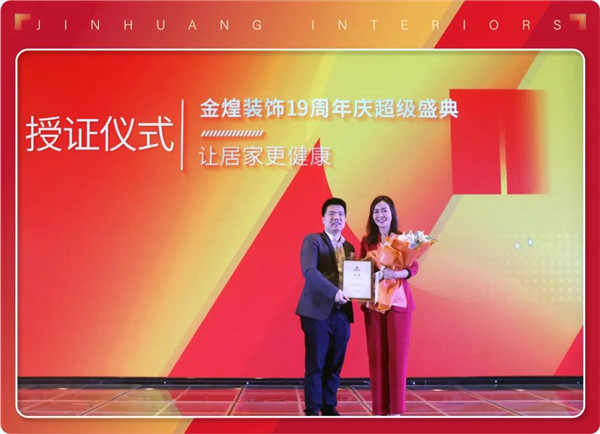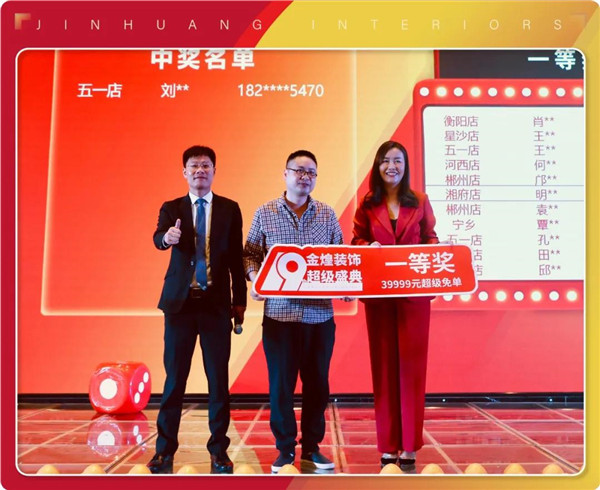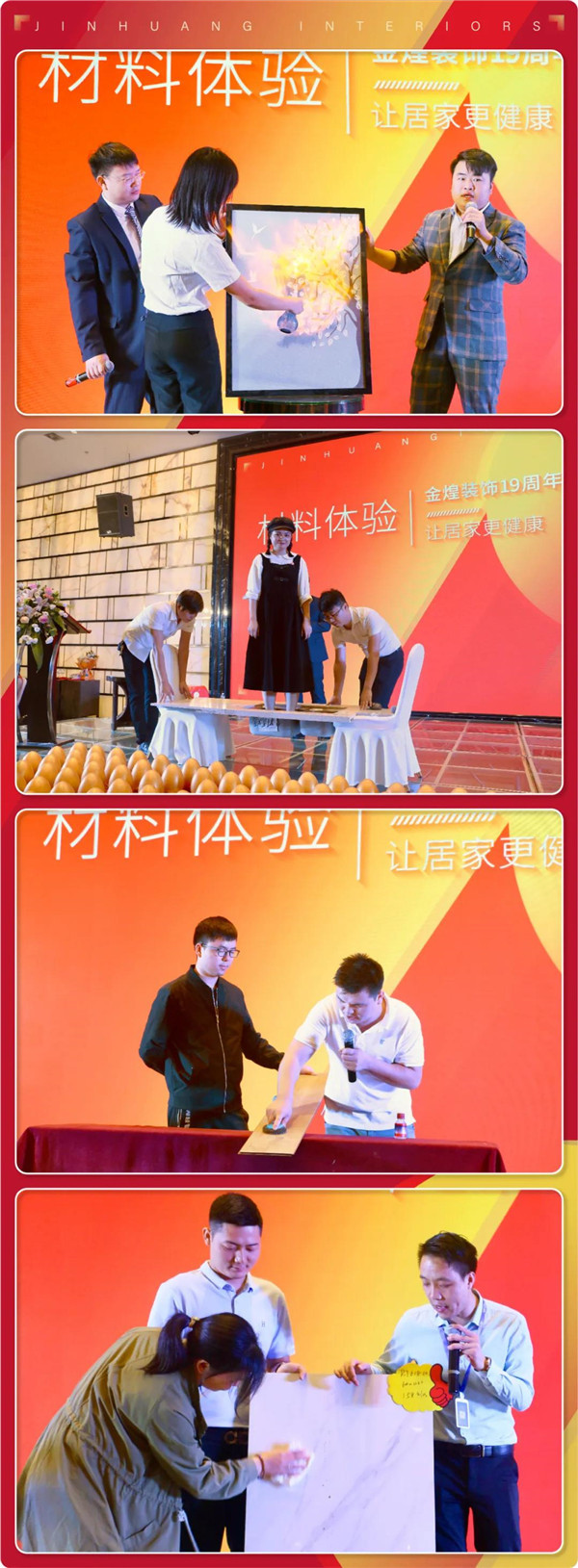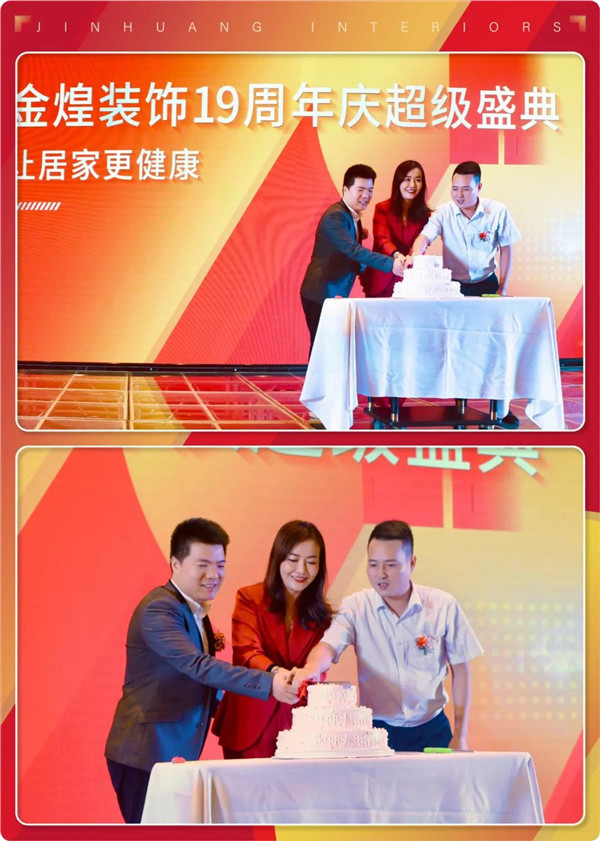|

# 长沙装修公司19周年庆完美落幕,金煌再启新征程

19年卓越品质铸造辉煌

19年诚信服务百万家庭2020年9月20日，金煌装饰19周年庆超级盛典，在小天鹅戴斯大酒店隆重举行，到场的重量级嘉宾有：金煌装饰集团副董事长廖成名先生、金煌装饰集团总经理罗勤先生、金煌装饰形象代言人/湖南卫视主持人刘梦娜女士、圣象地板湖南区域总经理姚利辉先生、欧派橱柜家装部总经理王卫先生、诺贝尔副总经理刘俊卿先生、蓝天豚副总经理唐焯先生。同时来自金煌的500位新老业主朋友们也欢聚一堂，共同度过了金煌19周年的生日会！这场超级盛典上，金煌装饰集团副董事长廖成名先生发布了“金煌19周年品牌发展战略”，分享了金煌装饰绿色健康整装标准体系的升级计划。绿色健康理念、绿色健康工程、绿色健康服务三大板块，将是未来金煌整装新升级的重中之重。2020年，新时代下金煌绿色整装家装服务将以全新的面貌，为广大装修业主带来超乎想象的家装体验。金煌装饰集团总经理罗勤先生代表集团总部，在超级盛典上对金煌装饰的一路历程，做了简短精炼的总结发言：作为家装行业绿色整装领军品牌，金煌19年来一直以严苛的标准、先进的管理、优质的产品、领先的工艺、环保的材料，为每一户家庭打造温暖的家。在这个过程中，离不开每一个金煌人开拓进取的拼搏与奋斗。罗总代表公司对全体金煌人表达了感谢之情，他说：“团队是企业发展的动力，我们今天的成果是全体金煌人一步步稳扎稳打赢得的收获。”超级盛典的重磅嘉宾——金煌装饰形象代言人刘梦娜女士，此次隆重出席超级盛典活动是为了发布一个重磅消息。在2020年金煌装饰品牌战略的大框架下，绿色整装、健康住宅核心产品输出的前提下，刘梦娜女士出任“金煌装饰首席环保体验官”。首席环保体验官刘梦娜女士将对金煌装饰的最新环保材料、绿色整装样板房、环保标准工程等板块进行最前沿的体验与推广。在活动现场，刘梦娜女士讲述了她与金煌装饰之间的不解之缘。多年前，刘梦娜女士选择金煌装饰的装修服务，当时就对金煌的环保装修理念、服务态度、工艺水准记忆深刻。这么多年来，她家的房子依然完好如初。所以她不仅将自家后来的房子交由金煌装饰装修，更是向身边的亲朋好友同事积极推荐金煌装饰的绿色整装，从客户变为代言人、首席环保体验官，这正是因为刘梦娜女士对健康家装的注重，亲身体验了金煌的品质后，来自她发自内心的认可。超级盛典现场的500位VIP业主是金煌最重要的客人，拳拳盛意只为表达19年周年庆感恩回馈之意。大礼包、砸金蛋、大抽奖……应接不暇，金煌联合诺贝尔、欧派、圣象、蓝天豚等材料大牌一同推出专属礼遇。19周年庆作为金煌装饰年度最重要的活动，得到了新老客户的大力追捧。活动期间，金煌装饰为广大业主朋友送出了价值39999元的全房主材免单大礼以及各种优惠政策，力度之大、覆盖面之广为2020年之最。19周年庆的超级免单大奖由刘梦娜女士亲手抽出，在公证员的监督下，这个翘首以盼的惊喜终于诞生。周年庆盛典通过网络直播形式与全省各地州市金煌装饰分公司同步，各地的火爆场景通过网络直播感染着全省的金煌家人和业主朋友们，让“家人”同庆的氛围更加浓郁。蓝天豚硅藻泥、欧派橱柜、诺贝尔瓷砖、圣象地板等金煌装饰主材供应商的代表纷纷上台与业主互动，通过现场实验的形式让业主朋友亲眼目睹亲身体验，最直观的感受金煌家装服务的卓越品质。19年的历程，铸就了金煌装饰卓越的口碑、稳健的品牌。精于一行、勇于创新的金煌人奋斗拼搏，与时俱进，深耕家庭装修设计施工服务，强强联合，领跑行业。凡是过往，皆是序章！未来的路可能更长、更艰难，但我们相信，只要我们勇敢无畏、奋力向前，秉持“利他、合作、共享”的价值观，不断继往开来，我们的路必将越走越宽广。我们也一定能一一实现金煌装饰的初心与目标：“为更多的人打造健康环保的家”。

`声明：本文由入驻焦点开放平台的作者撰写，除焦点官方账号外，观点仅代表作者本人，不代表焦点立场错误信息举报电话： 400-099-0099，邮箱：jubao@vip.sohu.com，或点此进行意见反馈，或点此进行举报投诉。`A B C D E F G H J K L M N P Q R S T W X Y Z
A - B - C - D - E
• A
• 鞍山
• 安庆
• 安阳
• 安顺
• 安康
• 澳门
• B
• 北京
• 保定
• 包头
• 巴彦淖尔
• 本溪
• 蚌埠
• 亳州
• 滨州
• 北海
• 百色
• 巴中
• 毕节
• 保山
• 宝鸡
• 白银
• 巴州
• C
• 承德
• 沧州
• 长治
• 赤峰
• 朝阳
• 长春
• 常州
• 滁州
• 池州
• 长沙
• 常德
• 郴州
• 潮州
• 崇左
• 重庆
• 成都
• 楚雄
• 昌都
• 慈溪
• 常熟
• D
• 大同
• 大连
• 丹东
• 大庆
• 东营
• 德州
• 东莞
• 德阳
• 达州
• 大理
• 德宏
• 定西
• 儋州
• 东平
• E
• 鄂尔多斯
• 鄂州
• 恩施
F - G - H - I - J
• F
• 抚顺
• 阜新
• 阜阳
• 福州
• 抚州
• 佛山
• 防城港
• G
• 赣州
• 广州
• 桂林
• 贵港
• 广元
• 广安
• 贵阳
• 固原
• H
• 邯郸
• 衡水
• 呼和浩特
• 呼伦贝尔
• 葫芦岛
• 哈尔滨
• 黑河
• 淮安
• 杭州
• 湖州
• 合肥
• 淮南
• 淮北
• 黄山
• 菏泽
• 鹤壁
• 黄石
• 黄冈
• 衡阳
• 怀化
• 惠州
• 河源
• 贺州
• 河池
• 海口
• 红河
• 汉中
• 海东
• 怀来
• I
• J
• 晋中
• 锦州
• 吉林
• 鸡西
• 佳木斯
• 嘉兴
• 金华
• 景德镇
• 九江
• 吉安
• 济南
• 济宁
• 焦作
• 荆门
• 荆州
• 江门
• 揭阳
• 金昌
• 酒泉
• 嘉峪关
K - L - M - N - P
• K
• 开封
• 昆明
• 昆山
• L
• 廊坊
• 临汾
• 辽阳
• 连云港
• 丽水
• 六安
• 龙岩
• 莱芜
• 临沂
• 聊城
• 洛阳
• 漯河
• 娄底
• 柳州
• 来宾
• 泸州
• 乐山
• 六盘水
• 丽江
• 临沧
• 拉萨
• 林芝
• 兰州
• 陇南
• M
• 牡丹江
• 马鞍山
• 茂名
• 梅州
• 绵阳
• 眉山
• N
• 南京
• 南通
• 宁波
• 南平
• 宁德
• 南昌
• 南阳
• 南宁
• 内江
• 南充
• P
• 盘锦
• 莆田
• 平顶山
• 濮阳
• 攀枝花
• 普洱
• 平凉
Q - R - S - T - W
• Q
• 秦皇岛
• 齐齐哈尔
• 衢州
• 泉州
• 青岛
• 清远
• 钦州
• 黔南
• 曲靖
• 庆阳
• R
• 日照
• 日喀则
• S
• 石家庄
• 沈阳
• 双鸭山
• 绥化
• 上海
• 苏州
• 宿迁
• 绍兴
• 宿州
• 三明
• 上饶
• 三门峡
• 商丘
• 十堰
• 随州
• 邵阳
• 韶关
• 深圳
• 汕头
• 汕尾
• 三亚
• 三沙
• 遂宁
• 山南
• 商洛
• 石嘴山
• T
• 天津
• 唐山
• 太原
• 通辽
• 铁岭
• 泰州
• 台州
• 铜陵
• 泰安
• 铜仁
• 铜川
• 天水
• 天门
• W
• 乌海
• 乌兰察布
• 无锡
• 温州
• 芜湖
• 潍坊
• 威海
• 武汉
• 梧州
• 渭南
• 武威
• 吴忠
• 乌鲁木齐
X - Y - Z
• X
• 邢台
• 徐州
• 宣城
• 厦门
• 新乡
• 许昌
• 信阳
• 襄阳
• 孝感
• 咸宁
• 湘潭
• 湘西
• 西双版纳
• 西安
• 咸阳
• 西宁
• 仙桃
• 西昌
• Y
• 运城
• 营口
• 盐城
• 扬州
• 鹰潭
• 宜春
• 烟台
• 宜昌
• 岳阳
• 益阳
• 永州
• 阳江
• 云浮
• 玉林
• 宜宾
• 雅安
• 玉溪
• 延安
• 榆林
• 银川
• Z
• 张家口
• 镇江
• 舟山
• 漳州
• 淄博
• 枣庄
• 郑州
• 周口
• 驻马店
• 株洲
• 张家界
• 珠海
• 湛江
• 肇庆
• 中山
• 自贡
• 资阳
• 遵义
• 昭通
• 张掖
• 中卫

1室1厅1厨1卫1阳台

1
2
3
4
5

0
1
2

1

1

0
1
2
3报名成功，资料已提交审核A B C D E F G H J K L M N P Q R S T W X Y Z
A - B - C - D - E
• A
• 鞍山
• 安庆
• 安阳
• 安顺
• 安康
• 澳门
• B
• 北京
• 保定
• 包头
• 巴彦淖尔
• 本溪
• 蚌埠
• 亳州
• 滨州
• 北海
• 百色
• 巴中
• 毕节
• 保山
• 宝鸡
• 白银
• 巴州
• C
• 承德
• 沧州
• 长治
• 赤峰
• 朝阳
• 长春
• 常州
• 滁州
• 池州
• 长沙
• 常德
• 郴州
• 潮州
• 崇左
• 重庆
• 成都
• 楚雄
• 昌都
• 慈溪
• 常熟
• D
• 大同
• 大连
• 丹东
• 大庆
• 东营
• 德州
• 东莞
• 德阳
• 达州
• 大理
• 德宏
• 定西
• 儋州
• 东平
• E
• 鄂尔多斯
• 鄂州
• 恩施
F - G - H - I - J
• F
• 抚顺
• 阜新
• 阜阳
• 福州
• 抚州
• 佛山
• 防城港
• G
• 赣州
• 广州
• 桂林
• 贵港
• 广元
• 广安
• 贵阳
• 固原
• H
• 邯郸
• 衡水
• 呼和浩特
• 呼伦贝尔
• 葫芦岛
• 哈尔滨
• 黑河
• 淮安
• 杭州
• 湖州
• 合肥
• 淮南
• 淮北
• 黄山
• 菏泽
• 鹤壁
• 黄石
• 黄冈
• 衡阳
• 怀化
• 惠州
• 河源
• 贺州
• 河池
• 海口
• 红河
• 汉中
• 海东
• 怀来
• I
• J
• 晋中
• 锦州
• 吉林
• 鸡西
• 佳木斯
• 嘉兴
• 金华
• 景德镇
• 九江
• 吉安
• 济南
• 济宁
• 焦作
• 荆门
• 荆州
• 江门
• 揭阳
• 金昌
• 酒泉
• 嘉峪关
K - L - M - N - P
• K
• 开封
• 昆明
• 昆山
• L
• 廊坊
• 临汾
• 辽阳
• 连云港
• 丽水
• 六安
• 龙岩
• 莱芜
• 临沂
• 聊城
• 洛阳
• 漯河
• 娄底
• 柳州
• 来宾
• 泸州
• 乐山
• 六盘水
• 丽江
• 临沧
• 拉萨
• 林芝
• 兰州
• 陇南
• M
• 牡丹江
• 马鞍山
• 茂名
• 梅州
• 绵阳
• 眉山
• N
• 南京
• 南通
• 宁波
• 南平
• 宁德
• 南昌
• 南阳
• 南宁
• 内江
• 南充
• P
• 盘锦
• 莆田
• 平顶山
• 濮阳
• 攀枝花
• 普洱
• 平凉
Q - R - S - T - W
• Q
• 秦皇岛
• 齐齐哈尔
• 衢州
• 泉州
• 青岛
• 清远
• 钦州
• 黔南
• 曲靖
• 庆阳
• R
• 日照
• 日喀则
• S
• 石家庄
• 沈阳
• 双鸭山
• 绥化
• 上海
• 苏州
• 宿迁
• 绍兴
• 宿州
• 三明
• 上饶
• 三门峡
• 商丘
• 十堰
• 随州
• 邵阳
• 韶关
• 深圳
• 汕头
• 汕尾
• 三亚
• 三沙
• 遂宁
• 山南
• 商洛
• 石嘴山
• T
• 天津
• 唐山
• 太原
• 通辽
• 铁岭
• 泰州
• 台州
• 铜陵
• 泰安
• 铜仁
• 铜川
• 天水
• 天门
• W
• 乌海
• 乌兰察布
• 无锡
• 温州
• 芜湖
• 潍坊
• 威海
• 武汉
• 梧州
• 渭南
• 武威
• 吴忠
• 乌鲁木齐
X - Y - Z
• X
• 邢台
• 徐州
• 宣城
• 厦门
• 新乡
• 许昌
• 信阳
• 襄阳
• 孝感
• 咸宁
• 湘潭
• 湘西
• 西双版纳
• 西安
• 咸阳
• 西宁
• 仙桃
• 西昌
• Y
• 运城
• 营口
• 盐城
• 扬州
• 鹰潭
• 宜春
• 烟台
• 宜昌
• 岳阳
• 益阳
• 永州
• 阳江
• 云浮
• 玉林
• 宜宾
• 雅安
• 玉溪
• 延安
• 榆林
• 银川
• Z
• 张家口
• 镇江
• 舟山
• 漳州
• 淄博
• 枣庄
• 郑州
• 周口
• 驻马店
• 株洲
• 张家界
• 珠海
• 湛江
• 肇庆
• 中山
• 自贡
• 资阳
• 遵义
• 昭通
• 张掖
• 中卫• 手机• 分享
• 设计
免费设计
• 计算器
装修计算器
• 入驻
合作入驻
• 联系
联系我们
• 置顶
返回顶部